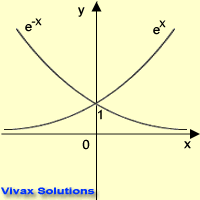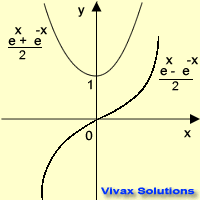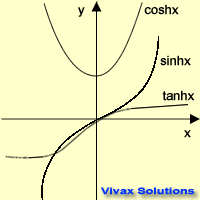# Mathematics

### Hyperbolic Functions

Functions that are defined in terms of the exponential function or its derivatives are called hyperbolic functions.The exponential function and its derivatives take these forms.

E.g

sinh x = (ex - e-x) / 2

cosh x = (ex + e-x) / 2

tanh x = sinh x / cosh x = (ex - e-x) / (ex + e-x)

In order to obtain the values of hyperbolic functions, the following table can be used.

 Enter 'x' here

Let's see the graphical representation of the three hyperbolic functions.Now, the basic properties of these functions can easily be derived:

E.g.1

cosh-x = cosh x
cosh x = (ex + e-x) / 2
cosh -x = (e(-x) + e-(-x)) / 2 = (ex + e-x) / 2 = cosh x

E.g.2

sinh -x = -sinh x
sinh x = (ex - e-x) / 2
sinh -x = (e(-x) - e-(-x)) / 2 = (e-x - ex) / 2 = - sinh x

E.g.3

tanh -x = -tanh x
tanh x = (ex - e-x) / (ex + e-x)
tanh -x = (e-x - e-(-x)) / (e-x + e-(-x)) = (e-x - ex) / (e-x + ex) = -tanh x

The functions can be extended to bring about the following identities.

1. cosh(a + b) = cosh(a)cosh(b) + sinh(a)sinh(b)
2. sinh(a + b) = sinh(a)cosh(b) + cosh(a)sinh(b)
3. cosh(a - b) = cosh(a)cosh(b) - sinh(a)sinh(b)
4. sinh(a - b) = sinh(a)cosh(b) - cosh(a)sinh(b)
5. tanh (a+b) = tanh a + tanh b /(1 + tanh a.tanh b)

Please work out the following questions to complement what you have just learnt.

1. Show that tanh x is an odd function.
2. Show that cosh2x - sinh2x = 1
3. Differentiate y = sinh x and y = cosh x
4. Prove that cosh x is even and sinh x is odd.
5. Find the point on y = cosh x curve, where the gradient is one.Maths is challenging; so is finding the right book. K A Stroud, in this book, cleverly managed to make all the major topics crystal clear with plenty of examples; popularity of the book speak for itself - 7th edition in print.

### Recommended - GCSE & iGCSEThis is the best book available for the new GCSE(9-1) specification and iGCSE: there are plenty of worked examples; a really good collection of problems for practising; every single topic is adequately covered; the topics are organized in a logical order.

### Recommended for A LevelThis is the best book that can be recommended for the new A Level - Edexcel board: it covers every single topic in detail;lots of worked examples; ample problems for practising; beautifully and clearly presented.